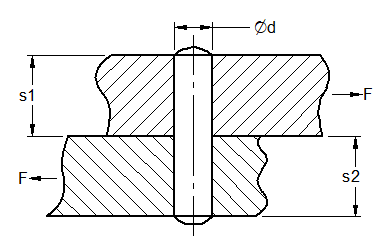Related Resources: calculators

### Dowel Pin Shear Load Stress Equations and Calculator

Pin, Dowel Design Length Shear Stress and Contact Pressure Check Equations and Calculator

The following equations can be used for wood dowels or other pin materials single shear design and analysis.Pin Dowel Shear Stress Equation:

τ = ( 4 · F ) / ( π · d2 ) ≤ τA

τA = su / fos

Contact Pressure Design Equation:

p1 = F / ( d · s1 ) ≤ p1A

p1A = su1 / fos

p2 = F / ( d · s2 ) ≤ p2A

p2A = s2 / fos

where:

F = Force applied (lbs, N),
τ = shear stress on dowel pin [psi, MPa],
τA = allowable shear stress [psi, MPa],
su = Shear or yield strength of dowel pin material [psi, MPa],
fos = Factor of safety for dowel pin and boards, typical 2 or greater,
d = pin diameter [in, mm],
p1 = pressure in the top board [psi, MPa],
p2 = pressure in the bottom board [psi, MPa],
p1A = allowable pressure in the top board [psi, MPa],
p2A = allowable pressure in the bottom board [psi, MPa],
s1 = thickness of the top board [in, mm],
s2 = thickness of the bottom board [in, mm].

Reference:

Kent's Machine Engineers Handbook, 12th Edition Design and Production, 1950 Colin Carmichael

Related web pages: